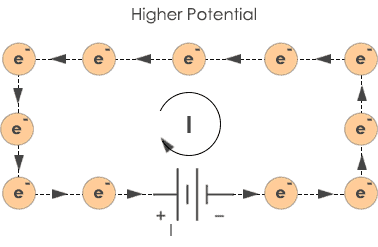# Drift Current Drift Velocity and Electron Mobility

As you all know that an electric conductor has free electrons inside them, due to movement of these free electrons, current is produced and when we give them energy via connecting battery, the electrons move towards higher potential.

Note that movement of electron is opposite to the direction of the current. Current flows from higher potential to lower while electrons are attracted towards higher potential and move in the opposite direction of the current.Imagine a piece of conductor and free electrons moving in them. Their motion is random and with no respect to their direction. Now, we apply an electric field across the conductor. Let us consider what is actually happening as the current flows through a conductor. The free electrons which are actually negatively charged particles will start moving towards the higher potential of the source connected across the conductor. In this stage, they do not lose their randomness of motion but starts their journey towards higher potential.

How randomness is maintained inside an electrically stressed conductor?

There are some free electrons inside a conductor in normal conditions. This free electrons move randomly inside the conductor, they collide with certain atoms bigger than them and in this process, they lose their kinetic energy.

Now, we connect a battery, the electrons will move towards the positive terminal of the battery and they get this energy to move via electric potential of the battery. In this movement, they collide with each other and other atoms bigger than them lose their kinetic energy. Also, they get the kinetic energy if a bigger atom collides with them, their acceleration increases in this case. So, they are again in motion due to the electric potential and gain kinetic energy but due to some collision, they again lose it.

So, randomness is maintained inside the conductor. If the electric field intensity is increased inside the conductor, the electrons are accelerated towards the positive terminal of the battery. Thus, in this way of losing and gaining kinetic energy randomness is maintained inside the conductor in the presence of electric field.

## Drift Current

You all know that due to the motion of electrons, current is produced. So, the current produced due to this drift movement of electrons is known as drift current. Let’s define it-The current due to the drift motion of electrons inside an electrically stressed conductor is called drift current.

## Drift Velocity

Electrons are charge carriers and have charge ‘q’ and move with velocity ‘v’. This velocity is known as drift velocity. Thus, the velocity of drift motion of electrons (charge carriers) inside an electrically stressed conductor is known as drift velocity.

The Formula for drift velocity is given by

V=I/nAq

Where ‘v’ is the drift velocity

‘n’ is the no. of charge carriers

‘A’ is the cross-sectional area

‘Q’ is the charge of charge carriers.

The motion of electrons can also be referred as electron mobility means ability of electron to move inside the conductor. If ‘v’ is the drift velocity and ‘E’ is the electric field intensity, then

v=µE

Where µ is the electron mobility.

## Current density

Current density is the amount of electric current flowing per unit area of the electrically stressed conductor. There is a relation between current density and drift velocity. We denote the current density as J.

J=I/A

And therefore, J=nev

Where ‘v’ is drift velocity.

Thus, in this way, we can relate current density and drift velocity and we can observe they are directly proportional to each other. More the drift velocity more would be the current density.

So, this is all you need to know about drift current, drift velocity, current density, and mobility of electrons inside the conductor. Drift motion of electrons is responsible for the flow of current and thus we can get the conducting property in the materials.